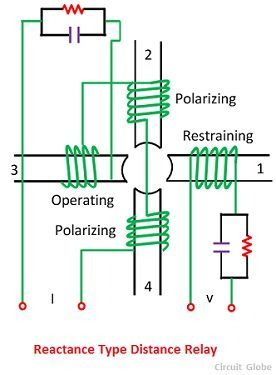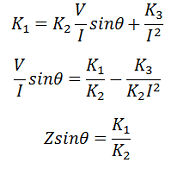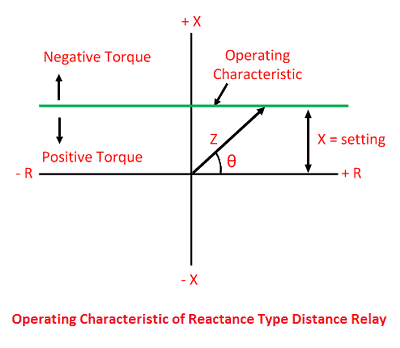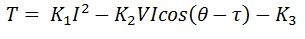# Reactance Relay

The reactance relay is a high-speed relay. This relay consists of two elements an overcurrent element and a current-voltage directional element. The current element developed positive torque and a current-voltage developed directional element which opposes the current element depending on the phase angle between current and voltage.

Reactance relay is an overcurrent relay with directional limitation. The directional element is arranged to develop maximum negative torque when its current lag behinds its voltage by 90°. The induction cup or double induction loop structures are best suited for actuating reactance type distance relays.

### Construction of Reactance Relay

A typical reactance relay using the induction cup structure is shown in the figure below. It has a four-pole structure carrying operating, polarizing, and restraining coils, as shown in the figure below. The operating torque is developed by the interaction of fluxes due to current carrying coils, i.e., the interaction of fluxes of 2, 3 and 4 and the restraining torque is produced by the interaction of fluxes due to poles 1, 2 and 4.The operating torque will be proportional to the square of the current while the restraining torque will be proportional to VI cos (Θ – 90°). The desired maximum torque angle is obtained with the help of resistance-capacitance circuits, as illustrated in the figure. If the control effect is indicated by –k3, the torque equation becomeswhere Θ, is defined as positive when I lag behind V. At the balance point net torque is zero, and henceThe spring control effect is neglected in the above equation, i.e., K3 = 0.

### Operating Characteristic of Reactance Relay

The operating characteristic of a reactance relay is shown in the figure below. X is the reactance of the protected line between the relay location and the fault point, and R is the resistance component of the impedance. The characteristic shows that the resistance component of the impedance has no consequence on the working of the relay, the relay reacts solely to the reactance component. The point below the operating characteristic is called the positive torque region.If the value of τ, in the general torque equation, expressed below is made any other 90º, a straight line characteristic will still be obtained, but it will not be parallel to R-axis. Such a relay is called an angle impedance relay.This type of relay is not capable of selecting whether the fault has taken place in the section where the relay is located, or it has taken place in the adjoining section when used on the transmission line. The directional unit used with the reactance relay will not be same as used with the impedance type relay because the restraining reactive volt-ampere, in that case, will be nearly equal to zero.

Therefore the reactance type distance relay needs a directional unit that is inoperative under load conditions. Reactance type relay is very suitable as a ground relay for ground fault because its reach is not affected by fault impedance.